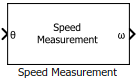# Speed Measurement

Compute speed from rotor angular position

• Library:
• Motor Control Blockset / Sensor Decoders

•## Description

The Speed Measurement block calculates the angular speed from the angular position of the rotor by calculating the change in the angular position with respect to time.

## Ports

### Input

expand all

Angular position of the rotor specified in either radians, degrees, or per-unit.

Data Types: `single` | `double` | `fixed point`

### Output

expand all

Angular speed that the block computes based on the angular position input.

Data Types: `single` | `double` | `fixed point`

## Parameters

expand all

The unit of the angular position `θ`.

The data type of the angular position input `θ`.

The speed calculation method used in the block. The selected method determines the range of the rotor speed that the block can measure.

These parameters change values according to the Speed calculation criteria parameter:

Parameter nameMaximum application speedSpeed ResolutionTime interval for speed calculation

Delays for speed calculation (number of samples)

`299`

`28``28`

Maximum measurable speed (RPM)

`1000`

`10344.8276``10713.2857`

Measurable speed resolution (RPM)

`4.6566e-07`

`4.9892e-06``4.9892e-06`

The fixed time interval (in seconds) between every two consecutive instances of block execution.

These parameters change values according to the Discrete step size (s) parameter value:

• Delays for speed calculation (number of samples)

• Maximum measurable speed (RPM)

• Measurable speed resolution (RPM)

The maximum rotor speed (in rotations per minute) that the block can measure.

These parameters change values according to the Maximum application speed (RPM) parameter value:

• Delays for speed calculation (number of samples)

• Maximum measurable speed (RPM)

• Measurable speed resolution (RPM)

#### Dependencies

To enable this parameter, set Speed calculation criteria to `Maximum application speed`.

The minimum value of change in the `θ` input per unit time that the block can detect.

These parameters change values according to the Speed Resolution (RPM) parameter value:

• Delays for speed calculation (number of samples)

• Maximum measurable speed (RPM)

• Measurable speed resolution (RPM)

#### Dependencies

To enable this parameter, set Speed calculation criteria to `Speed resolution`.

The number of samples of the angular position input that the block measures to compute the average position value.

These parameters change values according to the Delays for speed calculation (number of samples) parameter value:

• Maximum measurable speed (RPM)

• Measurable speed resolution (RPM)

#### Dependencies

To enable this parameter, set Speed calculation criteria to `Time interval for speed calculation`.

The absolute maximum speed that the block can measure.

This parameter is not configurable and uses a value that is internally computed using other parameters.

The minimum speed resolution that the block uses for speed computation. It is always less than or equal to Speed Resolution (RPM).

This parameter is not configurable and uses a value that is internally computed using other parameters.

Unit of the angular speed output.

The data type of the angular speed output `⍵`.

Note

The Speed Measurement block may occasionally display the warning message `'Wrap on overflow detected`.`'`